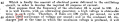# Old resonance frequency equation

#### captoro

Joined Jun 21, 2009
207

#### Attachments

Last edited by a moderator:

#### ericgibbs

Joined Jan 29, 2010
18,222
hi cap,
I don't see any attachments.E

#### captoro

Joined Jun 21, 2009
207
hi cap,
I don't see any attachments.E
It's up now...cheers

#### captoro

Joined Jun 21, 2009
207
Here is the image that didn't go up earlier

#### captoro

Joined Jun 21, 2009
207
I know it's from a patent. But mathematically..... How did he come up with it. It's different from a standard LC tank circuit formula.. and the 5033000...did he pill that out from his magic hat?. I'm plugging in the numbers on equation 5 and I am not arriving with the same results.

#### captoro

Joined Jun 21, 2009
207
I know it's from a patent. But mathematically..... How did he come up with it. It's different from a standard LC tank circuit formula.. and the 5033000...did he pill that out from his magic hat?. I'm plugging in the numbers on equation 5 and I am not arriving with the same results.
Actually . Where did HE get those equations?

#### ericgibbs

Joined Jan 29, 2010
18,222
•nsaspook and Danko

#### captoro

Joined Jun 21, 2009
207
hi cap,
Look at this clip,
E
View attachment 230517
I think I saw a link earlier to the fill pdf... Still don't get how they got 1/2pi equally to 503300....
Also in one of my first post. Equation 5. Don't know why there is a 1000 in the equation.

#### nsaspook

Joined Aug 27, 2009
12,293

#### Danko

Joined Nov 22, 2017
1,773
I think I saw a link earlier to the fill pdf... Still don't get how they got 1/2pi equally to 503300....
It depends on used units.
For example:

If L=1[H] and C=1[F] then
N=1/2π*√(1*1)=0.159 or N=0.159/√(1*1)=0.159,
so N=0.159/√(L[H]*C[F]).

If L=1000[mH] and C=1[F] then
N=1*√(1H/1mH)/2π*√(1000*1)=0.159 or N=5.033/√(1000*1)=0.159,
so N=5.033/√(L[mH]*C[F]).

L is in cm (0.000,000,001H) and C is in μF (0.000,001F) then
N=1*√(1,000,000,000*1,000,000)/2π*√(L[cm]*C[μF]),
so N=5,033,000/√(L[cm]*C[μF]).

------------------------------------------------------------------------
√((1H / 1cm) * (1F / 1μF)) / 2π = 5,033,000

Also in one of my first post. Equation 5. Don't know why there is a 1000 in the equation.
"1000", used in Equation 5, is circled below:Last edited:
•ericgibbs

#### sparky 1

Joined Nov 3, 2018
742
When a patent claim remains unproven the document containing math has a flaw.
The math can be legitimate and correct but the solution still has error because the variables deviate from defined standard.
For an invention to be both new and useful both working model and scientific method must be demonstrated.
Separating the math from machine is invalid and must remain together using the definitions in that document.
Math by itself also cannot be patented. The machine must always be considered in evaluating regardless of what math is used.
If later someone makes additional claims the patent must be evaluated with regard to appended claim. Nikola Tesla used methods to derive solutions and because of the unusual methods by todays understanding some confuse this as a scientific principal. People often read more into those documents than what was intended. The imagination naturally investigates other possibilities and must adhere to discipline never separating the math from the specific machine being described when evaluating a document.

Last edited:

#### captoro

Joined Jun 21, 2009
207
I can see the equation is a conversion from cm to henries, that part I get. But even on page 24 of the book , they assumes a value for L and C, and uses that as a constant later on. which seems odd to me. I tried the math, could not get it. I'll try again tonight

#### nsaspook

Joined Aug 27, 2009
12,293
I can see the equation is a conversion from cm to henries, that part I get. But even on page 24 of the book , they assumes a value for L and C, and uses that as a constant later on. which seems odd to me. I tried the math, could not get it. I'll try again tonight
http://web.mit.edu/8.02t/www/802TEAL3D/visualizations/coursenotes/modules/guide12.pdf
12-29

The magic number 5033 shows up here.

That number is just a shortcut for math with no physical properties other than a quick way to get a frequency from X powers of 10 sets of LC values. It's really no different from using RMS constant values in a calculation instead of the complete RMS calculation for X type of waveform.

https://en.wikipedia.org/wiki/Root_mean_square